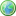Accessibility options:

# Simultaneous linear equations resources

Show me all resources applicable to

### Test Yourself (6)Diagnostic Test - Simultaneous equations
Diagnostic test for simultaneous equations. This resource is released under a Creative Commons license Attribution-Non-Commercial-No Derivative Works and the copyright is held by Skillbank Solutions Ltd.Exercise - Simultaneous equations
Online exercise on simultaneous equations. This resource is released under a Creative Commons license Attribution-Non-Commercial-No Derivative Works and the copyright is held by Skillbank Solutions Ltd.Maths EG
Computer-aided assessment of maths, stats and numeracy from GCSE to undergraduate level 2. These resources have been made available under a Creative Common licence by Martin Greenhow and Abdulrahman Kamavi, Brunel University.Simultaneous equations - Numbas
Two questions on solving systems of simultaneous equations. Numbas resources have been made available under a Creative Commons licence by Bill Foster and Christian Perfect, School of Mathematics & Statistics at Newcastle University.System of linear equations - Numbas
3 questions. First, two equations in two unknowns, second 3 equations in 3 unknowns, solved by Gauss elimination. The third two equations in 2 unknowns solved by putting into matrix form and finding the inverse of the coefficient matrix. Numbas resources have been made available under a Creative Commons licence by the School of Mathematics & Statistics at Newcastle University.Systems of linear equations - Numbas
3 questions. First, two equations in two unknowns; second 3 equations in 3 unknowns, solved by Gauss elimination. The third, two equations in 2 unknowns solved by putting into matrix form and finding the inverse of the coefficient matrix. Numbas resources have been made available under a Creative Commons licence by Bill Foster and Christian Perfect, School of Mathematics & Statistics at Newcastle University.

Website design by Pink Mayhem, Leicester